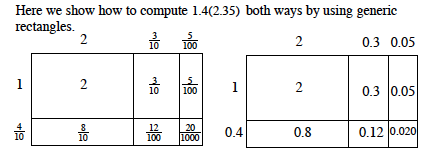Home > CC1MN > Chapter cc17 > Lesson cc17.1.2 > Problem7-23

7-23.

Calculate each of the following products. Homework Help ✎

1. $\frac { 2 } { 5 } \cdot \frac { 11 } { 14 }$

$\frac{2}{5}\cdot\frac{11}{14}= \frac{11}{35}$

1. $12\%\text{ of }32.8$

$0.12(32.8) = ?$

1. $(27.4)(0.02)$

Try using a generic rectangle like the ones pictured below.1. $2 \frac { 2 } { 3 } \cdot \frac { 3 } { 11 }$

This can be written as:

$\left ( \frac{8}{3} \right )\left ( \frac{3}{11} \right )$